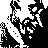1 CommentJan 8Liked by CyanneThere's another important detail about "Add with Carry" that I think the tutorial doesn't exactly mention: the carry flag is not just an *output*, set if overflow occurs, but it's also an *input*.Imagine doing a sum like "183 + 17" on paper, rather than in your head.- 3 + 7 is 10, so we write down 0 and carry the 1- 8 + 1 is 9, but we also have to add the 1 we carried before, which is another 10, so we write 0 and carry another 1- 1 + 0 is 1, but we also have to add the 1 we carried the second time, which is 2- and so we get the correct answer, 200"Add with Carry" adds the accumulator, the new values *and any previously carried 1*, clears the carry flag, then sets it again if the addition caused a new carry. That means you can use it to add 16, 24, 32 bit or even larger numbers as in the example above, treating each byte as if it were a single digit.When you do long-hand subtraction, sometimes you have the opposite problem: you can't (for example) subtract 6 from 4, so you have to borrow a 1 from the next higher decimal place. That's why "Subtract with Carry" uses the carry flag, although I'm not exactly sure *how* it uses it - left as an exercise for the reader. :)Expand full comment